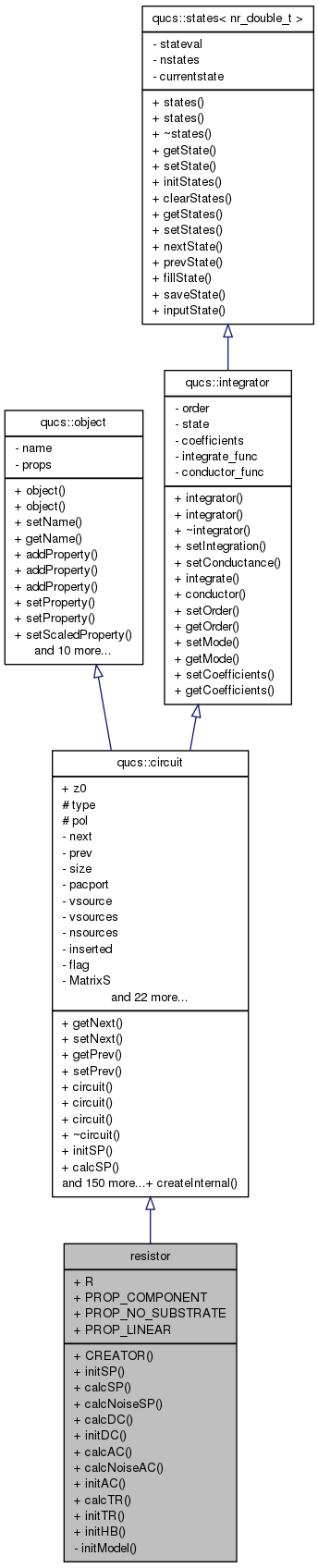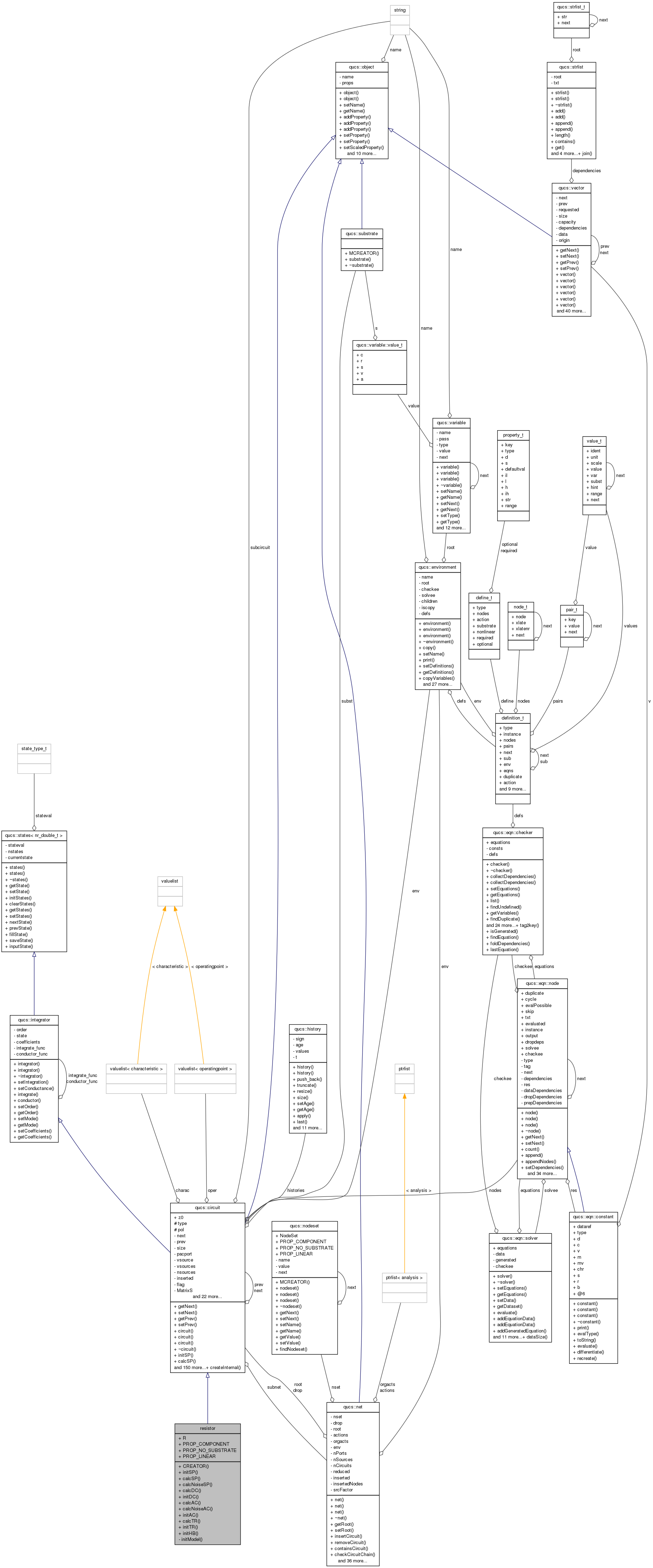Qucs-core  0.0.19
resistor Class Reference

`#include <resistor.h>`

Inheritance diagram for resistor:[legend]
Collaboration diagram for resistor:[legend]

## Public Member Functions

CREATOR (resistor)
void initSP (void)
placehoder for S-Parameter initialisation function
void calcSP (nr_double_t)
void calcNoiseSP (nr_double_t)
void calcDC (void)
void initDC (void)
void calcAC (nr_double_t)
void calcNoiseAC (nr_double_t)
void initAC (void)
void calcTR (nr_double_t)
void initTR (void)
void initHB (void)

## Data Fields

R
PROP_COMPONENT
PROP_NO_SUBSTRATE
PROP_LINEAR

## Private Member Functions

void initModel (void)

## Detailed Description

Definition at line 158 of file resistor.cpp.

## Member Function Documentation

 void resistor::calcAC ( nr_double_t ) ` [virtual]`

Reimplemented from qucs::circuit.

Definition at line 126 of file resistor.cpp.

 void resistor::calcDC ( void ) ` [virtual]`

Reimplemented from qucs::circuit.

Definition at line 111 of file resistor.cpp.

 void resistor::calcNoiseAC ( nr_double_t ) ` [virtual]`

Reimplemented from qucs::circuit.

Definition at line 61 of file resistor.cpp.

 void resistor::calcNoiseSP ( nr_double_t ) ` [virtual]`

Reimplemented from qucs::circuit.

Definition at line 52 of file resistor.cpp.

 void resistor::calcSP ( nr_double_t ) ` [virtual]`

Reimplemented from qucs::circuit.

Definition at line 43 of file resistor.cpp.

 void resistor::calcTR ( nr_double_t ) ` [virtual]`

Reimplemented from qucs::circuit.

Definition at line 134 of file resistor.cpp.

 resistor::CREATOR ( resistor )
 void resistor::initAC ( void ) ` [virtual]`

Reimplemented from qucs::circuit.

Definition at line 122 of file resistor.cpp.

 void resistor::initDC ( void ) ` [virtual]`

Reimplemented from qucs::circuit.

Definition at line 88 of file resistor.cpp.

 void resistor::initHB ( void ) ` [virtual]`

Reimplemented from qucs::circuit.

Definition at line 139 of file resistor.cpp.

 void resistor::initModel ( void ) ` [private]`

Definition at line 72 of file resistor.cpp.

 void resistor::initSP ( void ) ` [virtual]`

placehoder for S-Parameter initialisation function

Virtual function intended to be overridden by the inheiriting circuit element's S-Parameter initialisation function. initSP is called before commencing the simulation to set up the S-Parameter matrix.

Reimplemented from qucs::circuit.

Definition at line 38 of file resistor.cpp.

 void resistor::initTR ( void ) ` [virtual]`

Reimplemented from qucs::circuit.

Definition at line 130 of file resistor.cpp.

## Field Documentation

Definition at line 159 of file resistor.cpp.

Definition at line 159 of file resistor.cpp.

Definition at line 159 of file resistor.cpp.

Definition at line 159 of file resistor.cpp.

The documentation for this class was generated from the following files: